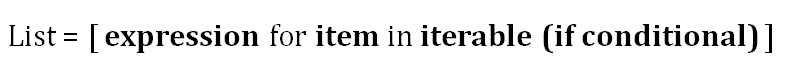1590457979

Python List: A Complete Tutorial to Lists, Loops and More !

Lists are one of the most powerful data types in Python. In this Python List Tutorial, you’ll learn how to work with lists while analyzing data about mobile apps. In this tutorial, we assume you know the very fundamentals of Python, including working with strings, integers, and floats.

#python list #python

Buddha Community1619518440

top 30 Python Tips and Tricks for Beginners

Welcome to my Blog , In this article, you are going to learn the top 10 python tips and tricks.

8) Check The Memory Usage Of An Object.

#python #python hacks tricks #python learning tips #python programming tricks #python tips #python tips and tricks #python tips and tricks advanced #python tips and tricks for beginners #python tips tricks and techniques #python tutorial #tips and tricks in python #tips to learn python #top 30 python tips and tricks for beginners1619510796

Lambda, Map, Filter functions in python

Welcome to my Blog, In this article, we will learn python lambda function, Map function, and filter function.

Lambda function in python: Lambda is a one line anonymous function and lambda takes any number of arguments but can only have one expression and python lambda syntax is

Syntax: x = lambda arguments : expression

Now i will show you some python lambda function examples:

#python #anonymous function python #filter function in python #lambda #lambda python 3 #map python #python filter #python filter lambda #python lambda #python lambda examples #python map1599099600

How to Use If-Else Statements and Loops in R – Dataquest

When we’re programming in R (or any other language, for that matter), we often want to control when and how particular parts of our code are executed. We can do that using control structures like if-else statements, for loops, and while loops.

Control structures are blocks of code that determine how other sections of code are executed based on specified parameters. You can think of these as a bit like the instructions a parent might give a child before leaving the house:

“If I’m not home by 8pm, make yourself dinner.”

Control structures set a condition and tell R what to do when that condition is met or not met. And unlike some kids, R will always do what we tell it to! You can learn more about control structures in the R documentation if you would like.

In this tutorial, we assume you’re familiar with basic data structures, and arithmetic operations in R.

Not quite there yet? Check out our Introductory R Programming course that’s part of our Data Analyst in R path. It’s free to start learning, there are no prerequisites, and there’s nothing to install — you can start learning in your browser right now.

install.packages(“Dataquest”)

Start learning R today with our Introduction to R course — no credit card required!

(This tutorial is based on our intermediate R programming course, so check that out as well! It’s interactive and will allow you to write and run code right in your browser.)

Comparison Operators in R

In order to use control structures, we need to create statements that will turn out to be either TRUE or FALSE. In the kids example above, the statement “It’s 8pm. Are my parents home yet?” yields TRUE (“Yes”) or FALSE (“No”). In R, the most fundamental way to evaluate something as TRUE or FALSE is through comparison operators.

Below are six essential comparison operators for working with control structures in R:

• == means equality. The statement x == a framed as a question means “Does the value of x equal the value of a?”
• != means “not equal”. The statement x == b means “Does the value of x not equal the value of b?”
• < means “less than”. The statement x < c means “Is the value of x less than the value of c?”
• <= means “less than or equal”. The statement x <= d means “Is the value of x less or equal to the value of d?”
• > means “greater than”. The statement x > e means “Is the value of x greater than the value of e?”
• >= means “greater than or equal”. The statement x >= f means “Is the value of xgreater than or equal to the value of f?”

#data science tutorials #beginner #for loop #for loops #if #if else #learn r #r #r tutorial #rstats #tutorial #tutorials #while loop #while loops1624591552

The anatomy of Python Lists

An easy guide to summarize the most common methods and operations regarding list manipulation in Python.

Python lists are a built-in type of data used to store items of any data type such as strings, integers, booleans, or any sort of objects, into a single variable.

Lists are created by enclosing one or multiple arbitrary comma-separated objects between square brackets.

Access values by index

#python-programming #python #tutorial #list-manipulation #python-list #the anatomy of python lists1624429860

How to Convert Loops to List Comprehension in Python

Do the same but faster

List comprehension is used for creating lists based on iterables. It can also be described as representing for and if loops with a simpler and more appealing syntax. List comprehensions are relatively faster than for loops.

The syntax of a list comprehension is actually easy to understand. However, when it comes to complex and nested operations, it might get a little tricky to figure out how to structure a list comprehension.

In such cases, writing the loop version first makes it easier to write the code for the list comprehension. We will go over several examples that demonstrate how to convert a loop-wise syntax to a list comprehension.Basic structure of list comprehension (image by author)

Let’s start with a simple example. We have a list of 5 integers and want to create a list that contains the squares of each item. Following is the for loop that performs this operation.

lst_a = [1, 2, 3, 4, 5]

lst_b = []
for i in lst_a:
lst_b.append(i**2)
print(lst_b)
[1, 4, 9, 16, 25]

#python #programming #how to convert loops to list comprehension in python #convert loops #list comprehension #how to convert loops to list comprehension